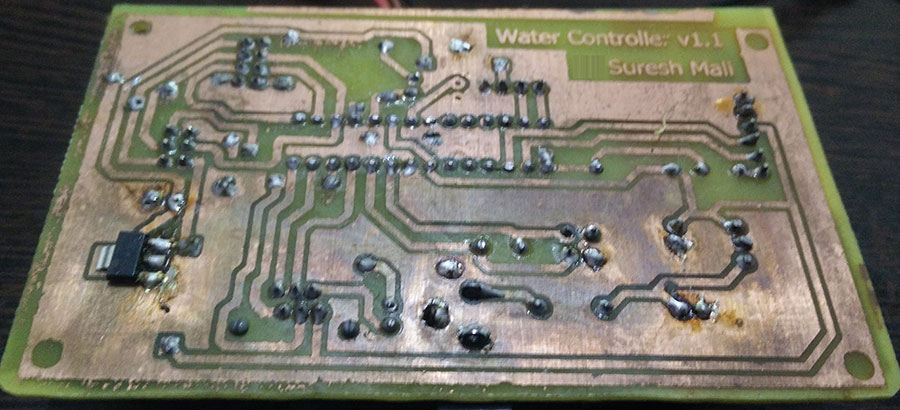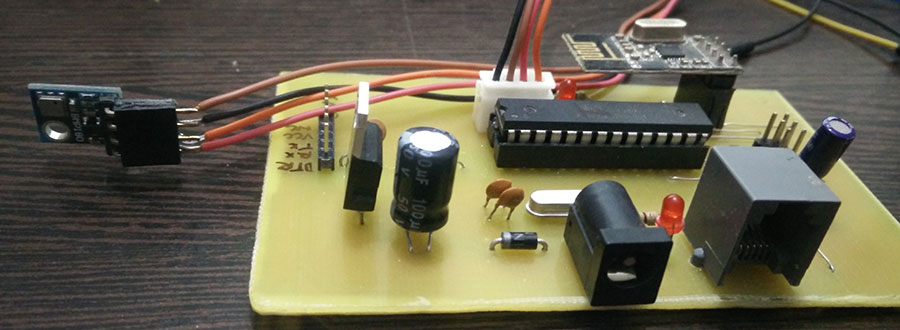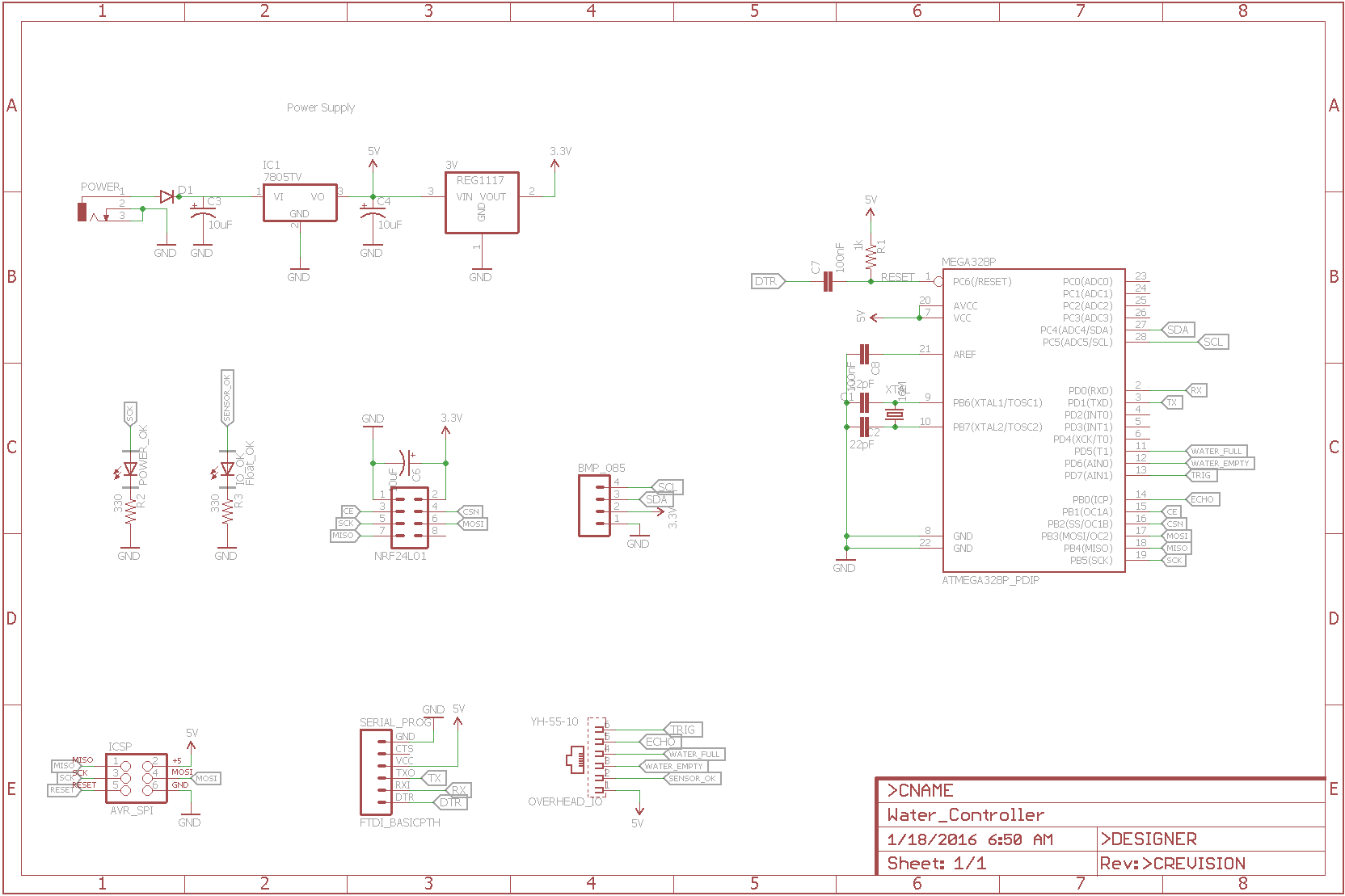# Pressure Sensor Node send fails.

• I have been trying to build a sensor node for Temp+Baro, and have been unsuccessful since last week. The message fails
Below is the output from Serial Monitor

``````req id
send: 255-255-1-0 s=255,c=3,t=3,pt=0,l=0,sg=0,st=fail:
sensor started, id=255, parent=1, distance=2
req id
send: 255-255-1-0 s=255,c=3,t=3,pt=0,l=0,sg=0,st=fail:
req id
send: 255-255-1-0 s=255,c=3,t=3,pt=0,l=0,sg=0,st=fail:
req id
send: 255-255-1-0 s=255,c=3,t=3,pt=0,l=0,sg=0,st=fail:
req id
send: 255-255-1-0 s=255,c=3,t=3,pt=0,l=0,sg=0,st=fail:
Temperature = 18.10 *C
Pressure = 1074.38 hPa
Forecast = unknown
req id
send: 255-255-1-0 s=255,c=3,t=3,pt=0,l=0,sg=0,st=fail:
req id
send: 255-255-1-0 s=255,c=3,t=3,pt=0,l=0,sg=0,st=fail:
req id
send: 255-255-1-0 s=255,c=3,t=3,pt=0,l=0,sg=0,st=fail:
Temperature = 17.90 *C
Pressure = 1074.37 hPa
Forecast = unknown
req id
send: 255-255-1-0 s=255,c=3,t=3,pt=0,l=0,sg=0,st=fail:
req id
send: 255-255-1-0 s=255,c=3,t=3,pt=0,l=0,sg=0,st=fail:
Temperature = 17.80 *C
Pressure = 1074.46 hPa
Forecast = unknown
req id
send: 255-255-1-0 s=255,c=3,t=3,pt=0,l=0,sg=0,st=fail:
req id
send: 255-255-1-0 s=255,c=3,t=3,pt=0,l=0,sg=0,st=fail:
``````

I have researched the forum a lot and tried the below, but no success.

• Added 10uF caps to both Node and Gateway. Also tried with 100uF caps.
• Cleared EEPROM.
• Tried different power source

I have one more node with Relay and its working fine. So i guess the problem should be with the node. The node is a custom built board with Optiboot. I worked fine a couple of times and now I wont work.Log file for MYSController when I had the MYSBootloader and tried updating FW using OTA. 0_1453856213616_MySensors_20160127-053410.log

I am unable to figure out the problem and seek assistance from the experienced gurus out there.

Appreciate and help I can get.

• I have also tried other sketch on same node and they work fine.

• Is your controller setup to hand out ID's to the sensor nodes?
What if you set a fixed ID?

• @ericvdb I tried with MYSController. Set Assign ID to Auto, also tried settings up static ID, but does not seem to work.

• Can you simply connect your baro sensor to relay node and see if something happens? Can you exchange hw/sw with the working (relay) node in any other way? Are both the working node and this one connecting to your repeater node 1 (parent = 1)?

1

3

7

92

2

3

2

3